Guru

# Question 1. If O is the centre of the circle, find the value of x in each of the following figures (using the given information):

• 1

This is circle based question from Chapter name- circles
Topic – Angle properties of circles
Chapter number- 15

In this question we have been given the figure of circle with certain information about the angles and we have to find the value of x.

ICSE Avichal publication
Understanding ICSE Mathematics
Question no 1, exercise 15.2

Share

1. Solution:
From the figure
(i) ABCD is a cyclic quadrilateral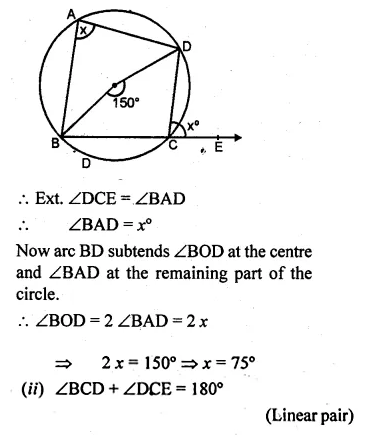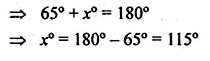• 3
2. Solution:

From the figure

(i) ABCD is a cyclic quadrilateral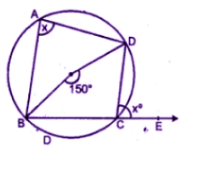Now arc BD subtends ∠BOD at the center

And ∠BAD at the remaining part of the circle.

∠BOD = 2 ∠BAD = 2 x

2 x = 150(x = 750)

(ii) ∠BCD + ∠DCE = 180o

(Linear pair)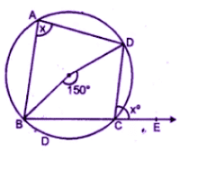∠BCD + 80= 180o

∠BCD = 180– 80= 100o

Arc BAD subtends reflex ∠BOD at the

Centre and ∠BCD at the remaining part of the circle

Reflex ∠BOD = 2 ∠BCD

X= 2 × 100= 200o

(iii) In ∆ACB,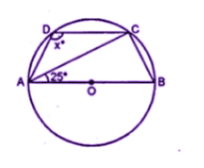∠CAB + ∠ABC + ∠ACB = 180o

(Angles of a triangle)

But
∠ACB = 90o

((Angles of a semicircle)

25+ 90+ ∠ABC = 180o

=115+ ∠ABC = 180o

∠ABC = 180– 1150=65o